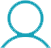#### Package Includes

• PHYSICS
• VECTOR (PKS SIR)
• VECTOR LECTURE-1
• VECTOR L2 AND L3 PART-1
• VECTOR L2 AND L3 PART-2
• VECTOR L2 AND L3 PART-3
• VECTOR LECTURE-4
• VECTOR LECTURE-5
• VECTOR LECTURE-6
• VECTOR L7 AND L8 PART-1 (CRQ.Q.17,19,20,21,27,28)
• VECTOR L7 AND L8 PART-2 (CRQ.Q.17,19,20,21,27,28)
• VECTOR L7 AND L8 PART-3 (CRQ.Q.17,19,20,21,27,28)
• VECTOR LECTURE-9
• CALCULUS (AA SIR)
• CALCULUS L1(AN INTRODUCTION TO TRIGONOMETRY)
• CALCULUS L2 PART 1(AN INTRODUCTION TO TRIGONOMETRY)
• CALCULUS L2 PART 2(AN INTRODUCTION TO TRIGONOMETRY)
• CALCULUS LECTURE 3 (AN INTRODUCTION TO TRIGONOMETRY)
• CALCULUS LECTURE 4 (Equations and Graphs of straight line)
• CALCULUS LECTURE 5 (Equations and graphs of straight line)
• CALCULUS LECTURE 6 (Equations and graphs of straight line Ques 1 and 2a)
• CALCULUS L7(EQUATIONS AND GRAPHS OF STRAIGHT LINE Q.2 (B) )
• CALCULUS L8 (AN INTRODUCTION TO MOTION OF OBJECT)
• CALCULUS L9ANDL10
• CALCULUS L11
• CALCULUS L12
• CALCULUS L13
• CALCULUS L14
• CALCULUS LECTURE 15(Concepts of Differentiation)
• CALCULUS LECTURE 16(Concepts of Differentiation)
• CALCULUS L17 (Mathematical operations with differentiation with CRQ question no. 11 and 12(a))
• CALCULUS L18 (Quotient rule of differentiation with CRQ questions 12(b) and 13)
• CALCULUS L19 Chain rule of differentiation
• CALCULUS L 20 " Chain rule of differentiation with CRQ question 14"
• CHEMISTRY
• BOCI (YG SIR)
• BOC-I LECTURE-1
• BOC-I LECTURE-2
• BOCI LECTURE 3
• BOC-I LECTURE 4 (CRQ.Q.7,8,9)
• BOCII (YG SIR)
• BOC-II LECTURE 1 (CRQ.Q.1,6,19)
• BOC-II L2 AND L3 PART-I (CRQ.Q)
• BOC-II L2 AND L3 PART-2 (CRQ.Q)
• BOC-II L2 AND L3 PART-3 (CRQ.Q)
• BOCII LECTURE 4 (CRQ.Q.9)
• BOC II L5 AND L6 PART-1 (CRQ.Q.)
• BOC II L5 AND L6 PART-2 (CRQ.Q.)
• BOC II L5 AND L6 PART-3 (CRQ.Q.)
• ASI (SKC SIR)
• AS-I LECTURE -1 (THEORY)
• AS LECTURE -2 (THEORY)
• AS LECTURE -3 (THEORY)
• AS LECTURE 4 (theory)
• AS LECTURE-5 (CRQ.Q.)
• AS LECTURE-6 (CRQ.Q.)
• ASLECTURE-7 (CRQ.Q.)
• AS LECTURE-8 (CRQ.Q.)
• AS LECTURE -9 (CRQ.Q.4,9,10,11,27,28)
• AS L10 AND L11 PART-1 (CRQ.Q.29)
• AS L10 AND L11 PART-2 (CRQ.Q.29)
• AS L10 AND L11 PART-3 (CRQ.Q.29)
• AS L12 AND L13 PART-1 (CRQ.Q.6)
• AS L12 AND L13 PART-2 (CRQ.Q.6)
• AS L12 AND L13 PART-3 (CRQ.Q.6)
• AS LECTURE 14
• AS LECTURE 15 (CRQ Q. 5,32,43)
• AS LECTURE 16 (CRQ Q.)
• AS L17 AND L18 PART1 (CRQ Q.34,35)
• AS L17 AND L18 PART2 (CRQ Q.34,35)
• AS L17 AND L18 PART3 (CRQ Q.34,35)
• AS LECTURE 19
• AS LECTURE 20 (CRQ.Q.)
• AS L21 AND L22 PART1 (CRQ Q.19,20,44,45)
• AS L21 AND L22 PART2 (CRQ Q.19,20,44,45)
• AS L21 AND L22 PART3 (CRQ Q.19,20,44,45)
• AS L23 AND L24 PART1 (THEORY)
• AS L23 AND L24 PART2 (THEORY)
• AS L23 AND L24 PART3 (THEORY)
• AS LECTURE 25
• AS LECTURE 26 (CRQ Q.22.23.24.25.12.13.31)
• AS LECTURE 27
• PT (ANB SIR)
• PT LECTURE 1 (CRQ Q.1)
• PT LECTURE 2 (CRQ Q.2)
• PT LECTURE 3
• PT L4 AND L5 PART-1
• PT L4 AND L5 PART-2
• PT L4 AND L5 PART-3
• PT L6 AND L7 PART-1 (CRQ.Q.12 to 15, 27)
• PT L6 AND L7 PART-2 (CRQ.Q.12 to 15, 27)
• PT L6 AND L7 PART-3 (CRQ.Q.12 to 15, 27)
• PT L8 AND L9 PART-1
• PT L8 AND L9 PART-2
• PT L8 AND L9 PART-3
• PT L10 AND L11 PART-1 (CRQ.Q.19.20.21.22.24.25.26.28.30.7.8.9)
• PT L10 AND L11 PART-2 (CRQ.Q.19.20.21.22.24.25.26.28.30.7.8.9)
• PT L10 AND L11 PART-3 (CRQ.Q.19.20.21.22.24.25.26.28.30.7.8.9)
• MATHS
• LOGRITHM (MR SIR)
• LOGARITHM LECTURE 1 (PART1)
• LOGARITHM LECTURE 1 (PART2)
• LOGARITHM LECTURE 1 (PART3)
• LOGARITHM LECTURE 2 (PART1)
• LOGARITHM LECTURE 2 (PART2)
• LOGARITHM LECTURE 2 (PART3)
• LOGARITHM LECTURE 3 (PART1)
• LOGARITHM LECTURE 3 (PART2)
• PNC (MR SIR)
• PNC LECTURE 1
• PNC LECTURE 2
• PNC LECTURE 3
• PNC LECTURE 4
• PNC LECTURE 5
• PNC LECTURE 6
• PNC LECTURE 7
• PNC LECTURE 8 PART1
• PNC LECTURE 8 PART2
• PNC LECTURE 9 PART1
• PNC LECTURE 9 PART2
• PNC LECTURE 10 PART1
• PNC LECTURE 10 PART2
• PNC LECTURE 11
• SET THEORY (MAC SIR)
• SET THEORY LECTURE-1 (CRQ.Q. 1 & 2)
• SET THEORY LECTURE-2 (CRQ.Q.)
• SET THEORY LECTURE -3 (CRQ.Q.5 (A,B) 7 (A TO H))
• SET THEORY LECTURE -4 (CRQ.Q.8,10)
• SET THEORY LECTURE -5 (CRQ.Q. 9 11 12 13 14 19(all))
• PMI (MAC SIR)
• PMI LECTURE-1 (CRQ.Q.1.2.34.5.6.7.8)
• PMI LECTURE-2 (CRQ.Q.)
• LINEAR INEQUALITIES (MAC SIR)
• LINEAR INEQUALITIES LECTURE -1 (CRQ.Q. 1)
• LINEAR INEQUALITIES L2 AND L3 PART-1 (CRQ.Q.2 to 12 & 14,15,17)
• LINEAR INEQUALITIES L2 AND L3 PART-2 (CRQ.Q.2 to 12 & 14,15,17)
• LINEAR INEQUALITIES L2 AND L3 PART-3 (CRQ.Q.2 to 12 & 14,15,17)
• APGPHP (MAC SIR)
• APGPHP LECTURE 1 (CRQ.Q.1,2,3,4)
• APGPHP LECTURE -2
• APGPHP L3 AND L4 PART-1 (CRQ.Q.6,7,13,15,18,19,20,21,59)
• APGPHP L3 AND L4 PART-2 (CRQ.Q.6,7,13,15,18,19,20,21,59)
• APGPHP L3 AND L4 PART-3 (CRQ.Q.6,7,13,15,18,19,20,21,59)
• APGPHP L5 AND L6 PART 1 (CRQ.Q.22,23,24,26,27,28,29,30,31,32,33)
• APGPHP L5 AND L6 PART 2 (CRQ.Q.22,23,24,26,27,28,29,30,31,32,33)
• APGPHP L5 AND L6 PART 3 (CRQ.Q.22,23,24,26,27,28,29,30,31,32,33)
• APGPHP L7 AND L8 PART-1
• APGPHP L7 AND L8 PART-2
• APGPHP LECTURE 9 (CRQ Q.45-49,51,53,61)
• APGPHP LECTURE 10

₹ 125000

Do you have coupon code?

Apply